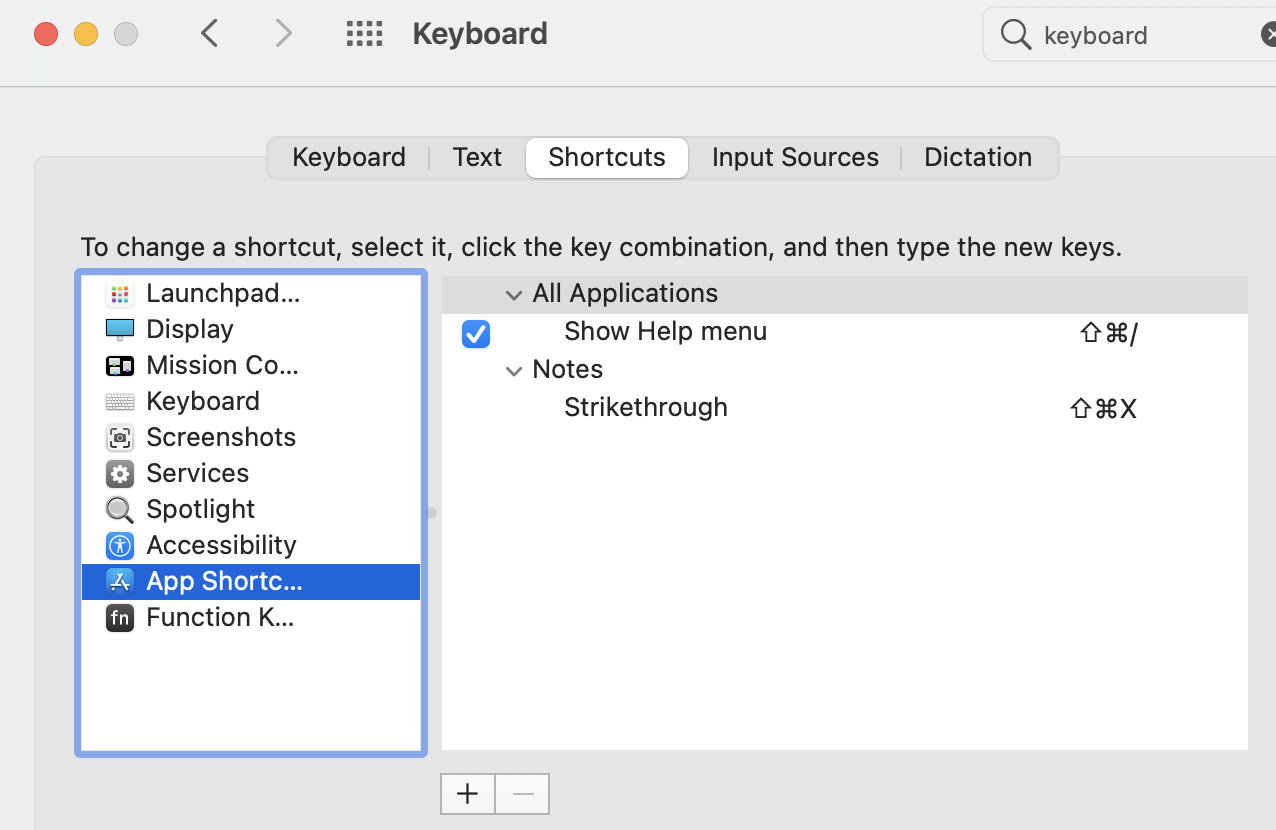### Practical examples on MS Excel date functions

MS Excel has an amazing set of Date and Time functions. By tweaking these functions, we can create a complex and useful formula. I am trying to list down a few practical examples particularly on the Date part, which will be very useful for a moderate to advanced Excel user.

How to find the end of the month for a given date

Note that EOMONTH will return a serial number. You have to change the format to date format.

=EOMONTH(TODAY(),0)

=EOMONTH(DATE(2018,10,10),0)

How to find the number of days remaining in a month

This is useful for interest calculation on first and last month on any type of loan repayment schedule.

Use DAYS, EOMONTH, and DATE functions.

=DAYS(EOMONTH(TODAY(),0),DATE(2018,10,10))

Number of days remaining in this month or current month

=DAYS(EOMONTH(TODAY(),0),TODAY())

Find the number of months between two dates

In other words, find the date difference in terms of months. DATEDIF is one of the very useful formulas in MS Excel. It will take 2 parameters, the first parameter is the date and the second parameter is the unit. A unit may be Y, M, or D each constitutes Years Months and Days.

=DATEDIF(TODAY(), DATE(2019,1,10), "M")
=DATEDIF(TODAY(), DATE(2019,1,10), "Y")
=DATEDIF(TODAY(), DATE(2019,1,10), "D")

If you want to calculate the non-cumulative difference use these options.

"MD" - Ignores the Year and Month.
"YD"  - Ignores the Year
"YM" - Ignores the Year

How to construct a date using Day, Month and Year

DATE formula returns a date value. Takes 3 parameters - day, month, and year. This formula is useful whenever you want to construct a date from different formats or from a text value. Before using this formula to extract date value from a text, you need to parse and get the individual DAY, MONTH, and YEAR values. I will discuss more on this later

Syntax:

=DATE(YEAR, MONTH, DAY)

=DATE(2018,10,10)

A practical scenario where the DATE function is useful. You have the date but you only want to change the Month.

=DATE(YEAR(B2), 12, DAY(B2))

How to get the first and last day of a month

First day:

=DAY(EOMONTH(TODAY(),-1)+1)

The above formula finds the end of the month of the previous month and adds one day to the date and then returns the day of the date value.

Last Day:

It is very simple to find the last day of a month using EOMONTH function

=DAY(EOMONTH(TODAY(),-1))

EOMONTH takes 2 parameters, Date and Month. The month value will be positive for future dates and negative for past dates, 0 for the current month.

0   - for the current month
-1  - previous month
3   - the 3rd month from now

How to convert month number to month name

Using the TEXT function, the month name can be extracted from a date value. Note the different formatting options and outputs.

=TEXT(TODAY(),"mmmm")
=TEXT(TODAY(),"mm")
=TEXT(DATE(2018,1,1),"m")
=TEXT(DATE(2018,1,1),"mm")
=TEXT(DATE(2018,1,1),"mmm")
=TEXT(DATE(2018,1,1),"mmm")

How to get the numerical value of a month text

Suppose I have a month value in the string format, say 'October', and I want this in numerical equivalent value 10. There is no readily available excel formula to get the numerical value of this string. We can use the below trick to get the numerical month value

In excel there is a shorthand to enter a date. By entering 1oct and then by pressing the enter key, we can get the date 1st October of the current year. Using this technique I will construct a formula to get the numerical value of text month value

=MONTH(1&LEFT(B24,3))
=MONTH(1&B25)
 =MONTH(1&MID(B26,6,3))How to get a Day, Month or a Year of a given date

It is very simple to extract a day, month, or a year from the date value. Just use the built-in Excel functions as shown below. These functions alone may not be that useful, but whenever you need to write a complex formula, these functions will be very helpful

=MONTH(TODAY())
=YEAR(TODAY())
=DAY(TODAY())

Convert any text into date format

If you have a date in a different format or the date is represented as text then is difficult to use that for any calculation purpose. First, you need to convert them into proper date format. You have to use different methods or techniques in different situations. I am giving a few examples here.

### Apple MacBook Notes strike through Menu option and Setting Keyboard shortcut.

Apple Notes is the probably most widely used App for taking notes on Apple MacBook or Apple Mac in general. In this tutorial, I will show y...